# Important Quantitative Aptitude Questions for RBI Assistant & IBPS CLERK – Set 4

Dear Banking Aspirant,

The most sought exam of the year is coming and a day away and the IBPS CLERK Mains Examination in the next month. So an intense preparation has to be made to ace the Mains Examination 2017. So we have been providing Important hard Level Questions for the IBPS Clerk Mains Exam, IBPS SO Prelims Exam and also for the RBI Assistant Prelims Exam 2017.

Solving tougher problems will provide the best way to practice for the forthcoming examination. So solve these questions and prepare in a best way to ace the exam in the days to come.

In the following questions two equations I and II are given. You have to solve both the equations and given the answer.

Q.1)

I. 2x=18×2-11×3

II. y2=25

(a) x > y

(b) x < y

(c) x ≥ y

(d) x ≤ y

(e) x = y or Relationship cannot be determined.

In the following questions two equations I and II are given. You have to solve both the equations and given the answer.

Q.2)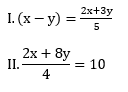(a) x > y

(b) x < y

(c) x ≥ y

(d) x ≤ y

(e) x = y or Relationship cannot be determined

In the following questions two equations I and II are given. You have to solve both the equations and given the answer.

Q.3)

I. x2-14x+33=0

II. y2-17y+60=0

(a) x > y

(b) x < y

(c) x ≥ y

(d) x ≤ y

(e) x = y or Relationship cannot be determined

In the following questions two equations I and II are given. You have to solve both the equations and given the answer.

Q.4)

I. x2+27x+170=

II. y2+5y-24=0

(a) x > y

(b) x < y

(c) x ≥ y

(d) x ≤ y

(e) x = y or Relationship cannot be determined

In the following questions two equations I and II are given. You have to solve both the equations and given the answer.

Q.5)

I. x2+12x-85=

II. y2-14y+45=0

(a) x > y

(b) x < y

(c) x ≥ y

(d) x ≤ y

(e) x = y or Relationship cannot be determined

In each of these questions two equation (I) and (II) are given. You have to solve both the equations and give answer.

(a) X > Y

(b) X < Y

(c) X ≥ Y

(d) X ≤ Y

(e) X = Y or the relationship between X and Y cannot be established

Q.6)

I.x2+16x+63=0

II.y2+15y+56=0

In each of these questions two equation (I) and (II) are given. You have to solve both the equations and give answer.

(a) X > Y

(b) X < Y

(c) X ≥ Y

(d) X ≤ Y

(e) X = Y or the relationship between X and Y cannot be established

Q.7)

I.2x2+16x+24=0

II.3y2-3y-18=0

In each of these questions two equation (I) and (II) are given. You have to solve both the equations and give answer.

(a) X > Y

(b) X < Y

(c) X ≥ Y

(d) X ≤ Y

(e) X = Y or the relationship between X and Y cannot be established

Q.8)

I.4x2-4x-8=0

II.5y2+25y+20=0

In each of these questions two equation (I) and (II) are given. You have to solve both the equations and give answer.

(a) X > Y

(b) X < Y

(c) X ≥ Y

(d) X ≤ Y

(e) X = Y or the relationship between X and Y cannot be established

Q.9)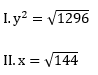In each of these questions two equation (I) and (II) are given. You have to solve both the equations and give answer.

(a) X > Y

(b) X < Y

(c) X ≥ Y

(d) X ≤ Y

(e) X = Y or the relationship between X and Y cannot be established

Q.10)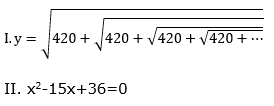Check the Answers for the above questions from the link given below

Explanation

Q.1) e

I. 2x=18×2-11×3

2x=36-33=3

x=1.5

II. y2=25

Relationship cannot be determined

Q.2) a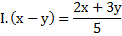5x-5y=2x+3y

3x-8y=0 ——-(1)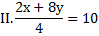2x+8y=40

x+4y=20 ——-(2)

Solve (1) and (2)

x= 8, y=3

x>y

Q.3) e

I. x2-14x+33=0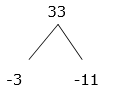x=3, 11

II. y2-17y+60=0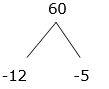y= 12, 5

Relationship cannot be determined.

Q.4) b

I. x2+27x+170=0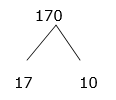x=-17, -10

II. y2+5y-24=0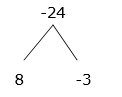y=-8, 3

x < y

Q.5) d

I. x2+12x-85=0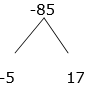x= 5, -17

II.y2-14y+45=0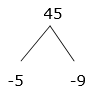y=5, 9

x ≤ y

Q.6) e

I.x2+16x+63=0

=>9+7=16; =>9×7=63

x=-7, -9

y2+15y+56=0

=>8+7=15; =>8×7=56

y=-7, -8

Relationship cannot be determined.

Q.7) d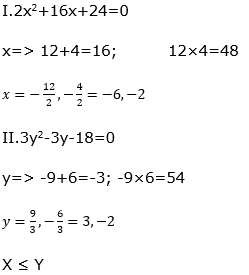Q.8) c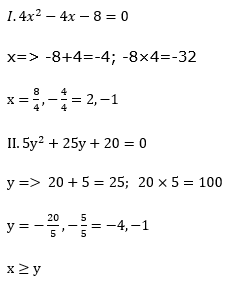Q.9) a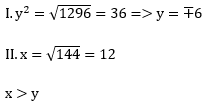Q.10) e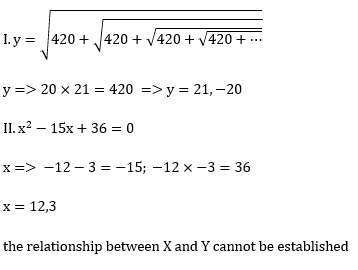You can check the other Important Reasoning Topics below here.